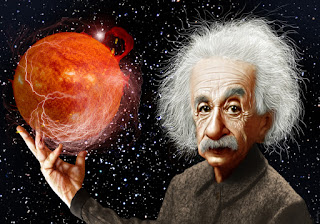## The SliderI published this post long ago in one of my old blog. This post become sooo popular that I thought to expand it with a few more examples. If you take any course on C/C++ programming, one of the common homework question is to print a diamond shape using asterisks (*) (or letters) and this question is asked in almost every freaking semester 😉 .

There are a lot of different ways to do that. First we will try solve it suing array-
#include<iostream>
using namespace std;
int main()

{
int i,j;
char arr;

for(i=0;i<5;i++)
{

for(j=0;j<5;j++)
{

arr[i][j]=' ';

}
}

arr='*';
arr='*';
arr='*';
arr='*';
arr='*';
arr='*';
arr='*';
arr='*';
arr='*';
arr='*';
arr='*';
arr='*';
arr='*';

for(i=0;i<5;i++)

{
cout<<endl;

for( j=0;j<5;j++)

{

cout<<" ";

cout<<arr[i][j];

}

}

return 0;

}



Explanation:
char arr;

creates a 6x6 array
for(i=0;i<5;i++)

{

for(j=0;j<5;j++)

Theses two for loops tells the program which array to leave a blank space.
arr='*';
arr='*';
arr='*';

....

....

These array specifies the box's position (column and row number) to put the asterisks. To create some other shape, just specify the position of the box and tell the program to put a asterisks there. Even though using array is relatively easy, this is kind of brute force approach. What if, you don't want to use array? and looking for a more general method? Well, here is another way to solve the problem-
#include<iostream>
#include<cstdlib>

using namespace std;

int main()
{

int i=0, j=0, NUM=3;

for(i=-NUM; i<=NUM; i++)

{

for(j=-NUM; j<=NUM; j++)

{

if( abs(i)+abs(j)<=NUM) // Change this condition

{

cout<<"*";

}

else { cout<<" ";}

}

cout<<endl;

}

return 0;

}


This code prints-
*
***
*****
*******
*****
***
*
Step by step Explanation:
int i=0, j=0, NUM=3;

We set a default value for the variables. If you need a bigger diamond, just change the NUM value.
for(i=-NUM; i<=NUM; i++)

This creates a matrix with 6 column and 6 rows.
for(j=-NUM; j<=NUM; j++)

{

if( abs(i)+abs(j)<=NUM)

{

cout<<"*";

}

else { cout<<" ";}

}

This for loop determines where to put the "*" and " " space using the if..else condition. And the function 'abs(i)' or 'abs(j) only takes the absolute value for 'i' and 'j'.
cout<<endl;

Goes to a new line after inserting all  the "*"s and " "s for a row. Say you need only right part of the diamond. You need to make a little change in the 'for loop' and 'if' condition:
for(i=-NUM; i<=NUM; i++)

{

for(j=0; j<=NUM; j++)

{

if( abs(i)+abs(j)<=NUM)

And it will print this-
*
**
***
****
***
**
*
Changing the code like this
for(i=-NUM; i<=0; i++)

{

for(j=0; j<=NUM; j++)

It will print this triangle-
*
**
***
****
But if you change the for loop like this-
for(i=0; i<=NUM; i++)

{

for(j=-NUM; j<=0; j++)

It will print the upside down triangle
****
***
**
*
Changing it this way-
for(i=-NUM; i<=NUM; i++)

{

for(j=0; j<=NUM; j++)

{

if( abs(i)+abs(j)>=NUM)

will result this shape:
****
***
**
*
**
***
****
Now let's say you want a diamond but you don't want the middle asterisks to appear. No worries, change the if condition like this-
if( abs(i)+abs(j)==NUM)

And you will get this-
*
*  *
*      *
*          *
*      *
*  *
*
Wait I haven’t done yet. Let's change the for loop like this- You can have more fun with it. Just change the ‘ if ‘ condition. You will get new designs 🙂. Wanna see???
if( abs(i)*abs(j)<= NUM)


***
***
*******
*******
*******
***
***
if( abs(i)==abs(j))


*          *
*      *
*  *
*
*  *
*      *
*          *
if( abs(i)==0||abs(j)==0)


*
*
*
*******
*
*
*
if( abs(i)>=abs(j))


*******
*****
***
*
***
*****
*******
if( abs(i)<=abs(j))

*          *
**      **
***  ***
*******
***  ***
**      **
*          *
Those who likes to use C code for printing diamond just change the header #include<iostream> to #include<stdio.h>, cout to printf and cin to scanf. Hope this post helps.Print shapes using asterisks in C/C++Reviewed by Dayeen on 1:44:00 AM Rating: 5Hello, my name is Jack Sparrow. I'm a 50 year old self-employed Pirate from the Caribbean.